Homework Help Question & Answers

# O ACIDS AND BASES Preparing a strong base solution with a given pH A chemist must...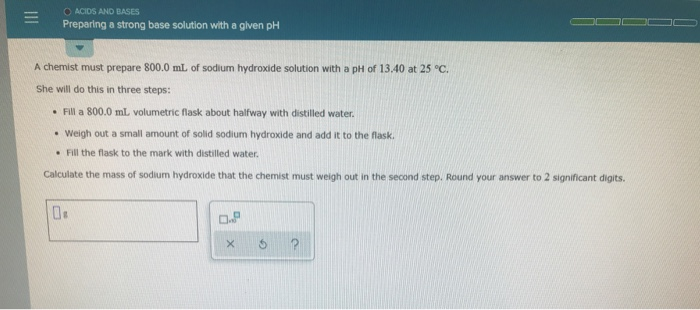O ACIDS AND BASES Preparing a strong base solution with a given pH A chemist must prepare 800.0 mL of sodium hydroxide solution with a pH of 13.40 at 25°C. She will do this in three steps: • Fill a 800.0 mL volumetric flask about halfway with distilled water. • Weigh out a small amount of solid sodium hydroxide and add it to the flask. • Fill the flask to the mark with distilled water. Calculate the mass of sodium hydroxide that the chemist must weigh out in the second step. Round your answer to 2 significant digits. X 5 ?

#### Homework Answers

Answer #1

1st find the concentration of NaOH

use:

pH = -log [H+]

13.4 = -log [H+]

[H+] = 3.981*10^-14 M

use:

[OH-] = Kw/[H+]

Kw is dissociation constant of water whose value is 1.0*10^-14 at 25 oC

[OH-] = (1.0*10^-14)/[H+]

[OH-] = (1.0*10^-14)/(3.981*10^-14)

[OH-] = 0.2512 M

So,

[NaOH] = 0.2512 M

volume , V = 8*10^2 mL

= 0.8 L

use:

number of mol,

n = Molarity * Volume

= 0.2512*0.8

= 0.201 mol

Molar mass of NaOH,

MM = 1*MM(Na) + 1*MM(O) + 1*MM(H)

= 1*22.99 + 1*16.0 + 1*1.008

= 39.998 g/mol

use:

mass of NaOH,

m = number of mol * molar mass

= 0.201 mol * 40 g/mol

= 8.038 g

Answer: 8.0 g

Know the answer?
Your Answer:

#### Post as a guest

Your Name:

What's your source?

#### Earn Coin

Coins can be redeemed for fabulous gifts.

Not the answer you're looking for? Ask your own homework help question. Our experts will answer your question WITHIN MINUTES for Free.
Similar Homework Help Questions
• ### A chemist must prepare 750.0 mL of sodium hydroxide solution with a pH of 13.70 at...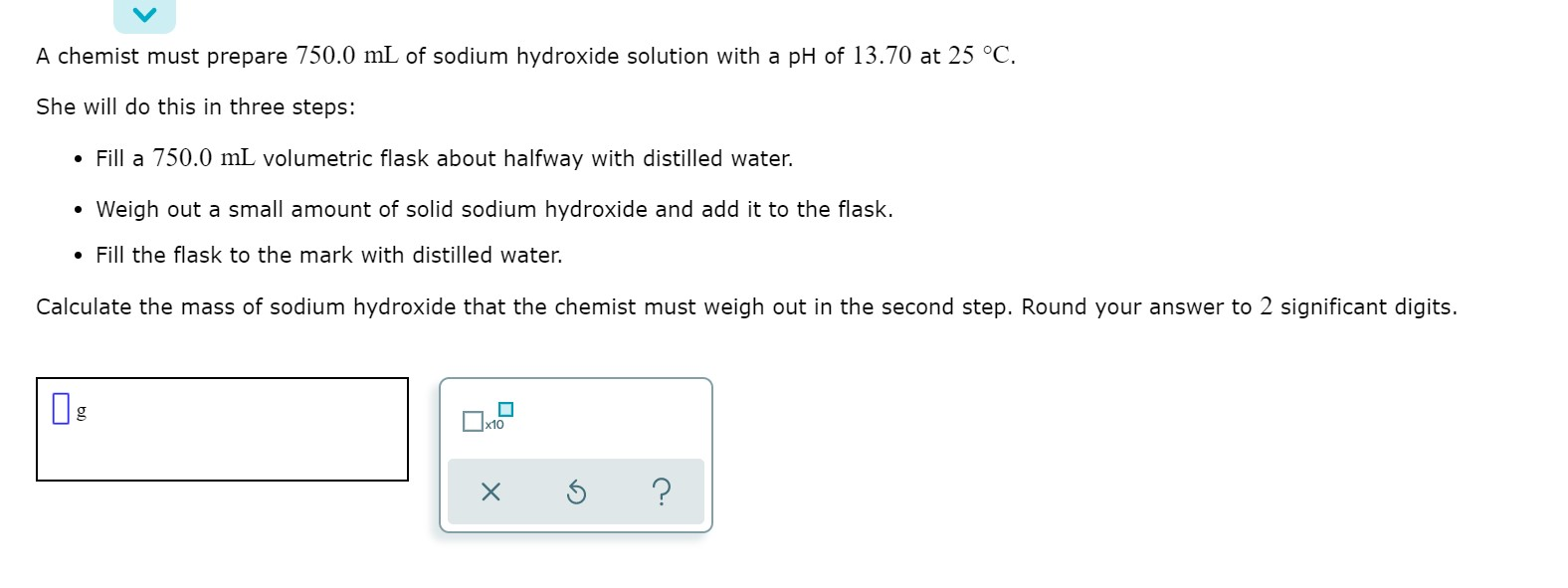A chemist must prepare 750.0 mL of sodium hydroxide solution with a pH of 13.70 at 25 °C. She will do this in three steps: • Fill a 750.0 mL volumetric flask about halfway with distilled water. • Weigh out a small amount of solid sodium hydroxide and add it to the flask. • Fill the flask to the mark with distilled water. Calculate the mass of sodium hydroxide that the chemist must weigh out in the second step. Round...

• ### A chemist must prepare 650.0mL of sodium hydroxide solution with a pH of 12.90 at 25°C....

A chemist must prepare 650.0mL of sodium hydroxide solution with a pH of 12.90 at 25°C. He will do this in three steps: Fill a 650.0mL volumetric flask about halfway with distilled water. Weigh out a small amount of solid sodium hydroxide and add it to the flask. Fill the flask to the mark with distilled water. Calculate the mass of sodium hydroxide that the chemist must weigh out in the second step. Round your answer to 2 significant digits.

• ### A chemist must prepare 850.0 mL of potassium hydroxide solution with a pH of 12.20 at...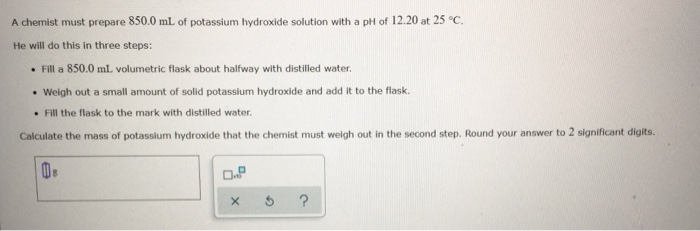A chemist must prepare 850.0 mL of potassium hydroxide solution with a pH of 12.20 at 25°C. He will do this in three steps: . Fill a 850.0 ml volumetric flask about halfway with distilled water. • Weigh out a small amount of solid potassium hydroxide and add it to the flask. • Fill the flask to the mark with distilled water. Calculate the mass of potassium Hydroxide that the chemist must weigh out in the second step. Round your...

• ### Objective Knowledge Check Question 14 A chemist must prepare 500.0 mL of potassium hydroxide solution with...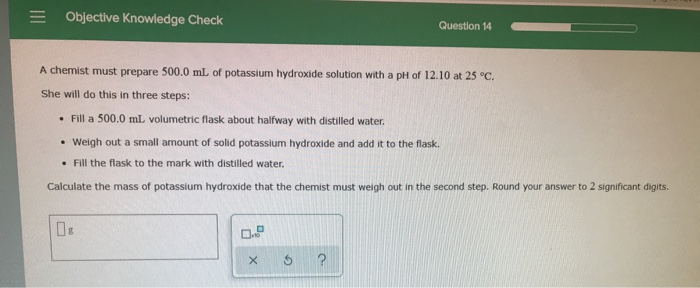Objective Knowledge Check Question 14 A chemist must prepare 500.0 mL of potassium hydroxide solution with a pH of 12.10 at 25 °C. She will do this in three steps: Fill a 500.0 mlL volumetric flask about halfway with distilled water Weigh out a small amount of solid potassium hydroxide and add it to the flask. Fill the flask to the mark with distilled water. Calculate the mass of potassium hydroxide that the chemist must weigh out in the second...

• ### O ACIDS AND BASES Calculating the pH of a strong base solution A chemist dissolves 287....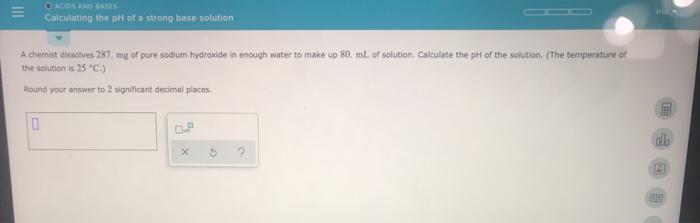O ACIDS AND BASES Calculating the pH of a strong base solution A chemist dissolves 287. mg of pure sodium hydroxide in enough water to make up 80. mL of solution. Calculate the pH of the solution. (The temperature of the solution is 25 °C.) Round your answer to 2 significant decimal places. X 5 ?

• ### O ACIDS AND BASES Calculating the pH of a strong base solution A chemist dissolves 402. mg of pure sodium hydroxide...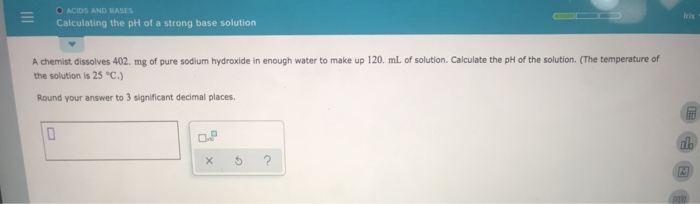O ACIDS AND BASES Calculating the pH of a strong base solution A chemist dissolves 402. mg of pure sodium hydroxide in enough water to make up 120 mL of solution. Calculate the pH of the solution. (The temperature of the solution is 25 °C.) Round your answer to 3 significant decimal places XS ?

• ### ACDS AND BASES Calculating the ph of a strong base solution A chemist dissolves 582. mg of pure sodium hydroxide in...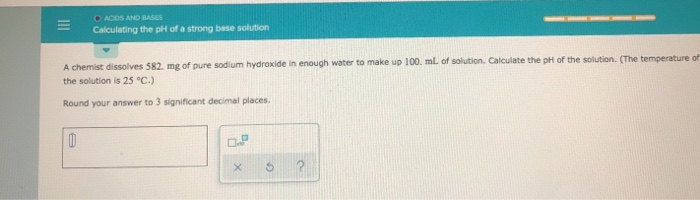ACDS AND BASES Calculating the ph of a strong base solution A chemist dissolves 582. mg of pure sodium hydroxide in enough water to make up 100 mL of solution. Calculate the pH of the solution. (The temperature of the solution is 25 °C.) Round your answer to 3 significant decimal places X

• ### OACIDS AND BASES Calculating the pH of a strong base solution A chemist dissolves 812, mg...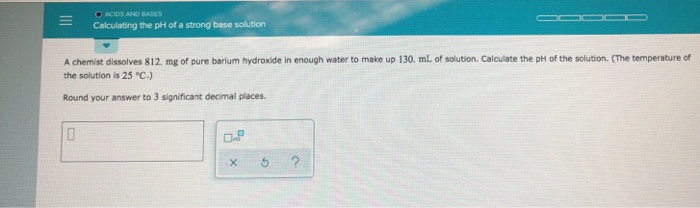OACIDS AND BASES Calculating the pH of a strong base solution A chemist dissolves 812, mg of pure barium hydroxide in enough water to make up 130. ml. of solution. Calculate the pH off the solution. (The temperature of the solution is 25 °C.) Round your answer to 3 significant decimal places. ? X

• ### O ACIDS AND BASES Calculating the pH of a weak acid titrated with a strong base...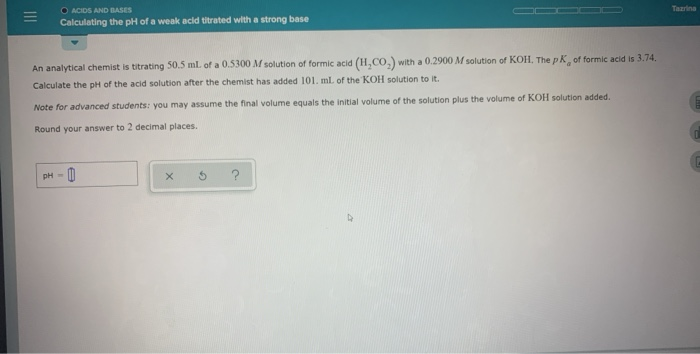O ACIDS AND BASES Calculating the pH of a weak acid titrated with a strong base An analytical Chemist is titrating 50.5 ml of a 0.5300 M solution of formic acid (H.CO.) with a 0.2900 M solution of KOH. The pk of formic acid is 3.74 Calculate the pH of the acid solution after the chemist has added 101. ml of the KOH solution to it. Note for advanced students you may assume the final volume equals the initial volume...

• ### O ACIDS AND BASES Calculating the pH of a weak acid Utrated with a strong base...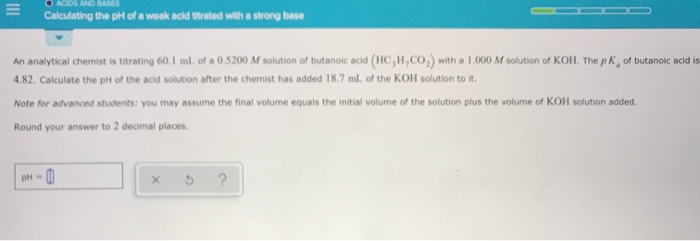O ACIDS AND BASES Calculating the pH of a weak acid Utrated with a strong base An analytical Chemist is titrating 60.1 ml of a 0.5200 M solution of butanolc acid (HC,H,CO) with a 1.000 M solution of KOH. The pk of butanoic acid is 4.82. Calculate the pH of the acid solution after the chemist has added 18.7 ml of the KOH solution to it. Note for advanced students you may assume the final volume equals the initial volume...